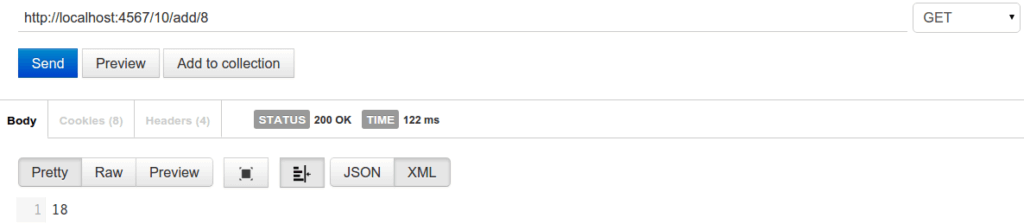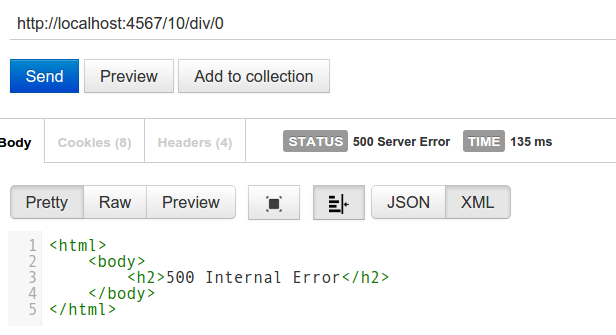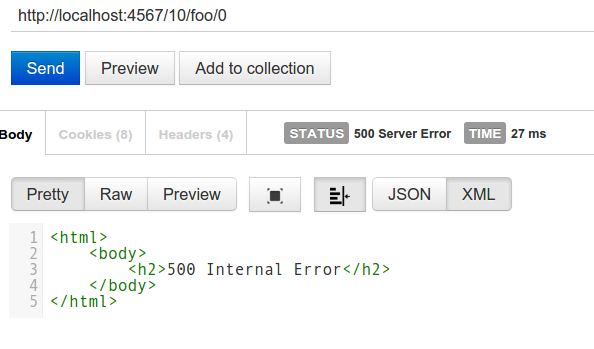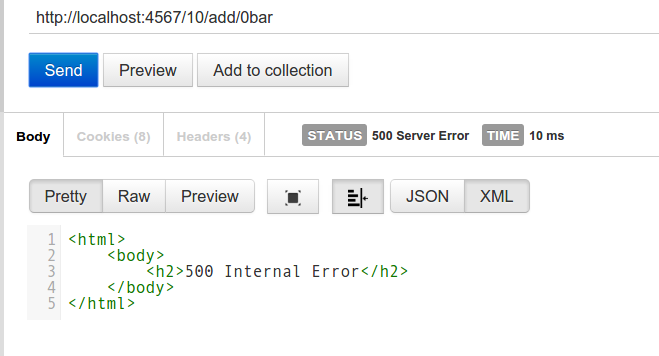# An Introduction to Spark, Your Next REST Framework for Java

### Learn how to set up Spark a web framework, and get your project going in Gradle, an overview of Spark, and explore tests.

· Integration Zone · Tutorial
Save
4.65K Views

Today we’re going to look into a refreshing, simple, nice and pragmatic framework for writing REST applications in Java. It will be so simple, it won’t even seem like Java at all.

We’re going to look into the Spark web framework. No, it’s not related to Apache Spark. Yes, it’s unfortunate that they share the same name.

I think the best way to understand this framework is to build a simple application, so we’ll build a simple service to perform mathematical operations.

We could use it like this:Note that the service is running on localhost at port 4567 and the resource requested is “/10/add/8”.

``````apply plugin: "java"
apply plugin: "idea"

sourceCompatibility = 1.8

repositories {
mavenCentral()
maven { url "https://oss.sonatype.org/content/repositories/snapshots/" }
maven { url "https://oss.sonatype.org/content/repositories/releases/" }
}

dependencies {
compile "com.javaslang:javaslang:2.0.0-RC1"
compile "com.sparkjava:spark-core:2.3"
compile "org.projectlombok:lombok:1.16.6"
testCompile group: 'junit', name: 'junit', version: '4.+'
}

classpath = sourceSets.main.runtimeClasspath
}``````

Now we can run:

• ./gradlew idea to generate an IntelliJ IDEA project
• ./gradlew test to run tests
• ./gradlew assemble to build the project
• ./gradlew launch to start our service

## Great. Now, Let’s Meet Spark

Do you think we can write a fully functional web service that performs basic mathematical operation in less than 25 lines of Java code? No way? Well, think again:

``````// imports omitted

class Calculator implements Route {

private Map<String, Function2<Long, Long, Long>> functions = ImmutableMap.of(
"add", (a, b) -> a + b,
"mul", (a, b) -> a * b,
"div", (a, b) -> a / b,
"sub", (a, b) -> a - b);

@Override
public Object handle(Request request, Response response) throws Exception {
long left = Long.parseLong(request.params(":left"));
String operatorName = request.params(":operator");
long right = Long.parseLong(request.params(":right"));
return functions.get(operatorName).apply(left, right);
}
}

public class SparkService {
public static void main(String[] args) {
get("/:left/:operator/:right", new Calculator());
}
}``````

In our main method, we just say that when we get a request which contains three parts (separated by slashes) we should use the Calculator route, which is our only route. A route in Spark is the unit which takes a request, processes it, and produces a response.

Our calculator is where the magic happens. It looks in the request for the parameters “left”, “operatorName” and “right”. Left and right are parsed as long values, while the operatorName is used to find the operation. For each operation, we have a Function (Function2<Long, Long>) which we then apply to our values (left and right). Cool, eh?

Function2 is an interface which comes from the Javaslang project.

You can now start the service (./gradlew launch, remember?) and play around.

The last time I checked Java was more verbose, redundant, slow… well, it is healing now.

## Ok, but What About Tests?

So Java can actually be quite concise, and as a Software Engineer I celebrate that for a minute or two, but shortly after I start to feel uneasy … this stuff has no tests! Worse than that, it doesn’t look testable at all. The logic is in our calculator class, but it takes a Request and produces a Response. I don’t want to instantiate a Request just to check if my Calculator works as intended. Let’s refactor a little:

``````class TestableCalculator implements Route {

private Map<String, Function2<Long, Long, Long>> functions = ImmutableMap.of(
"add", (a, b) -> a + b,
"mul", (a, b) -> a * b,
"div", (a, b) -> a / b,
"sub", (a, b) -> a - b);

public long calculate(String operatorName, long left, long right) {
return functions.get(operatorName).apply(left, right);
}

@Override
public Object handle(Request request, Response response) throws Exception {
long left = Long.parseLong(request.params(":left"));
String operatorName = request.params(":operator");
long right = Long.parseLong(request.params(":right"));
return calculate(operatorName, left, right);
}
}``````

We just separate the plumbing (taking the values out of the request) from the logic and put it in its own method: calculate. Now we can test calculate.

``````public class TestableLogicCalculatorTest {

@Test
public void testLogic() {
assertEquals(-6, new TestableCalculator().calculate("sub", 7, 13));
assertEquals(3, new TestableCalculator().calculate("mul", 3, 1));
assertEquals(0, new TestableCalculator().calculate("div", 0, 7));
}

@Test(expected = ArithmeticException.class)
public void testInvalidInputs() {
assertEquals(0, new TestableCalculator().calculate("div", 0, 0));
}

}``````

I feel better now: our tests prove that this stuff works. Sure, it will throw an exception if we try to divide by zero, but that’s how it is.

What does that mean for the user, though?It means this: a 500. And what happens if the user tries to use an operation which does not exist?What if the values are not proper numbers?Ok, this doesn’t seem very professional. Let’s fix it.

## Error Handling, Functional Style

To fix two of the cases we just have to use one feature of Spark: we can match specific exceptions to specific routes. Our routes will produce a meaningful HTTP status code and a proper message.

``````public class SparkService {
public static void main(String[] args) {
exception(NumberFormatException.class, (e, req, res) -> res.status(404));
exception(ArithmeticException.class, (e, req, res) -> {
res.status(400);
res.body("This does not seem like a good idea");
});
get("/:left/:operator/:right", new ReallyTestableCalculator());
}
}``````

We have still to handle the case of a non-existent operation, and this is something we are going to do in ReallyTestableCalculator.

To do so, we’ll use a typical function pattern: we’ll return an Either. An Either is a collection which can have either a left or a right value. The left typically represents some sort of information about an error, like an error code or an error message. If nothing goes wrong the Either will contain a right value, which could be all sort of stuff. In our case we will return an Error (a class we defined) if the operation cannot be executed, otherwise we will return the result of the operation in a Long. So we will return an Either<Error, Long>.

``````package me.tomassetti.javaadvent.calculators;

import javaslang.Function2;
import javaslang.Tuple2;
import javaslang.collection.Map;
import javaslang.collection.HashMap;
import javaslang.control.Either;
import spark.Request;
import spark.Response;
import spark.Route;

public class ReallyTestableCalculator implements Route {

private static final int NOT_FOUND = 404;

private Map<String, Function2<Long, Long, Long>> functions = HashMap.ofAll(
new Tuple2<>("add", (a, b) -> a + b),
new Tuple2<>("mul", (a, b) -> a * b),
new Tuple2<>("div", (a, b) -> a / b),
new Tuple2<>("sub", (a, b) -> a - b));

public Either<Error, Long> calculate(String operatorName, long left, long right) {
Either<Error, Long> unknownOp = Either.<Error, Long>left(new Error(NOT_FOUND, "Unknown math operation"));
return functions.get(operatorName).map(f -> Either.<Error, Long>right(f.apply(left, right)))
.orElse(unknownOp);
}

@Override
public Object handle(Request request, Response response) throws Exception {
long left = Long.parseLong(request.params(":left"));
String operatorName = request.params(":operator");
long right = Long.parseLong(request.params(":right"));
Either<Error, Long> res =  calculate(operatorName, left, right);
if (res.isRight()) {
return res.get();
} else {
response.status(res.left().get().getHttpCode());
return null;
}
}
}``````

Let’s test this:

``````package me.tomassetti.javaadvent;

import javaslang.control.Either;
import org.junit.Test;

import static org.junit.Assert.assertEquals;

public class ReallyTestableLogicCalculatorTest {

@Test
public void testLogic() {
assertEquals(Either.right(-6L), new ReallyTestableCalculator().calculate("sub", 7, 13));
assertEquals(Either.right(3L), new ReallyTestableCalculator().calculate("mul", 3, 1));
assertEquals(Either.right(0L), new ReallyTestableCalculator().calculate("div", 0, 7));
}

@Test(expected = ArithmeticException.class)
public void testInvalidOperation() {
Either<me.tomassetti.javaadvent.calculators.Error, Long> res = new ReallyTestableCalculator().calculate("div", 0, 0);
assertEquals(true, res.isLeft());
assertEquals(400, res.left().get().getHttpCode());
}

@Test
public void testUnknownOperation() {
Either<me.tomassetti.javaadvent.calculators.Error, Long> res = new ReallyTestableCalculator().calculate("foo", 0, 0);
assertEquals(true, res.isLeft());
assertEquals(404, res.left().get().getHttpCode());
}

}``````

## The Result

We got a service that can be easily tested. It performs mathematical operations. It supports the four basic operations, but it could be easily extended to support more. Errors are handled and the appropriate HTTP codes are used: 400 for bad inputs and 404 for unknown operations or values.

## Conclusions

When I first saw Java 8 I was happy about the new features, but not very excited. However, after a few, months I am seeing new frameworks come up which are based on these new features and have the potential to really change how we program in Java. Stuff like Spark and Javaslang is making the difference. I think that now Java can remain simple and solid while becoming much more agile and productive.

You can find many more tutorials either on the Spark tutorials website.

This is a post I wrote for the Java Advent. It was initially published here.

Topics: